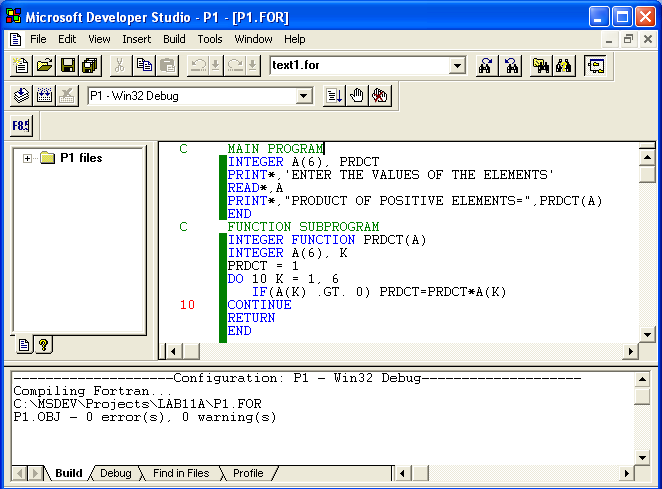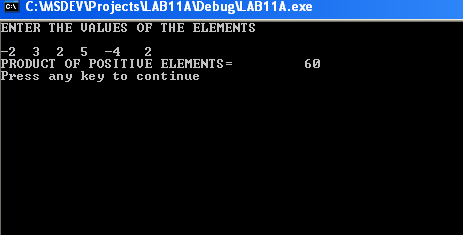ICS 101 - Computer programming

Lab # 11

(ONE-DIMENSIONAL ARRAYS) II

Objectives

•   ONE - DIMENSIONAL ARRAYS AND SUBPROGRAMS

One dimensional array can be passed to a subprogram or can be used locally within a subprogram. In both cases, the array  must be declared within the subprogram.

Example

Write a program, which reads a 1-D INTEGER array of size 6 it then prints the product of the positive elements (i.e. elements greater than zero) in the array. The product must be computed by an INTEGER function PRDCT invoked by the program.

Use the data: -2     3     2     5     -4     2

PRODUCT OF POSITIVE ELEMENTS =             60Following is the output of the program.Exercise 1

Write a function that takes a 1-D integer array and returns the average of its elements.

Then write a main program that reads a 1-D integer array of size 6, calls the Function

and prints the average of the elements of the 1-D array.

Use the data:

2          4          10          8          5          3

the average of the elements =          5.333333

Exercise 2

Write a program that reads the grades of a number of students that does not exceed 20.

The program should then pass these grades to a subroutine that computes and returns:

(1) the average of the grades (2) the maximum grade and (3) the count of grades below the average.

The values returned to the main program should then  be printed.

Use the data:

6

77.0        84.0        97.0        56.0        67.0        72.0